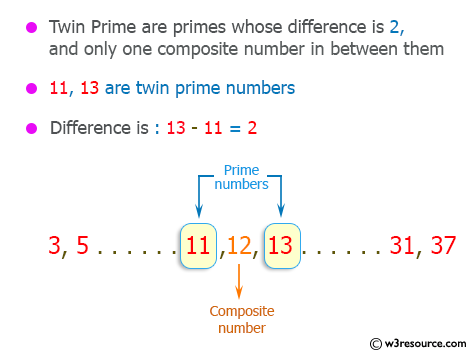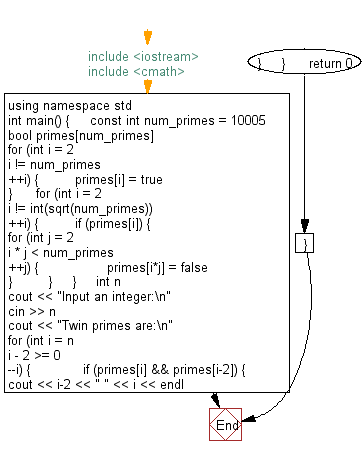﻿ C++ : Prints a twin prime which has the maximum size

# C++ Exercises: Prints a twin prime which has the maximum size among twin primes less than or equals to n

## C++ Basic: Exercise-62 with Solution

Write a C++ program that reads the integer n and prints a twin prime that has the maximum size among twin primes less than or equal to n.

According to wikipedia "A twin prime is a prime number that is either 2 less or 2 more than another prime number—for example, either member of the twin prime pair (41, 43). In other words, a twin prime is a prime that has a prime gap of two".

Pictorial Presentation:Sample Solution:

C++ Code :

``````#include <iostream>
#include <cmath>
using namespace std;

int main() {
const int num_primes = 10005;
bool primes[num_primes];
for (int i = 2; i != num_primes; ++i) {
primes[i] = true;
}

for (int i = 2; i != int(sqrt(num_primes)); ++i) {
if (primes[i]) {
for (int j = 2; i * j < num_primes; ++j) {
primes[i*j] = false;
}
}
}
int n;
cout << "Input an integer:\n";
cin >> n;
cout << "Twin primes are:\n";
for (int i = n; i - 2 >= 0; --i) {
if (primes[i] && primes[i-2]) {
cout << i-2 << " " << i << endl;
break;
}
}

return 0;
}
``````

Sample Output:

```Input an integer:
Twin primes are:
11 13
```

Flowchart:C++ Code Editor:

What is the difficulty level of this exercise?

﻿

## C++ Programming: Tips of the Day

Why is there no std::stou?

The most pat answer would be that the C library has no corresponding "strtou", and the C++11 string functions are all just thinly veiled wrappers around the C library functions: The std::sto* functions mirror strto*, and the std::to_string functions use sprintf.

Ref: https://bit.ly/3wtz2qA

We are closing our Disqus commenting system for some maintenanace issues. You may write to us at reach[at]yahoo[dot]com or visit us at Facebook